# NCERT Solutions for Class 9 Science Gravitation part 2## myCBSEguide App

CBSE, NCERT, JEE Main, NEET-UG, NDA, Exam Papers, Question Bank, NCERT Solutions, Exemplars, Revision Notes, Free Videos, MCQ Tests & more.

NCERT solutions for Science Gravitation## NCERT Class 9 Science Chapter wise Solutions

• 01 –  Matter in Our Surroundings
• 02 – Is Mattter Around us Pure
• 03 – Atoms and Molecules
• 04 – Structure of the Atom
• 05 – The Fundamental Unit of Life
• 06 – Tissues
• 07 – Diversity in Living Organisms
• 08 – Motion
• 09 – Force and Laws of Motion
• 10 – Gravitation
• 11 – Word and Energy
• 12 – Sound
• 13 – Why Do We Fall Ill
• 14 – Natural Resources
• 15 – Improvement in Food Resources

## NCERT Solutions for Class 9 Science Gravitation part 2

###### 1. How does the force of gravitation between two objects change when the distance between them is reduced to half?

Ans. The force of gravitation between two objects is inversely proportional to the square of the distance between them therefore the gravity will become four times if distance between them is reduced to half.

###### 2. Gravitational force acts on all objects in proportion to their masses. Why then, a heavy object does not fall faster than a light object?

Ans. In free fall of objects the acceleration in velocity due to gravity is independent of mass of those objects hence a heavy object does not fall faster than a light object.

###### 3. What is the magnitude of the gravitational force between the earth and a 1 kg object on its surface? (Mass of the earth is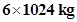and radius of the earth is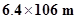.)

Ans.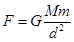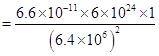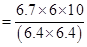= 9.81 N

NCERT Solutions for Class 9 Science Gravitation part 2

###### 4. The earth and the moon are attracted to each other by gravitational force. Does the earth attract the moon with a force that is greater or smaller or the same as the force with which the moon attracts the earth? Why?

Ans. The earth and the moon are attracted to each other by same gravitational force because for both of them formula to calculate force of attraction is the same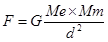d is also same for both.

###### 5. If the moon attracts the earth, why does the earth not move towards the moon?

Ans. Earth does not move towards moon because mass of moon is very small as compared to that of earth.

###### 6. What happens to the force between two objects, if

(i) the mass of one object is doubled?

(ii) the distance between the objects is doubled and tripled?

(iii) the masses of both objects are doubled?

Ans. (i) the force between two objects will be doubled.

(ii) the force between two objects will become 1/4th and 1/9th of the present force.

(iii) the force between two objects will become four times the present force.

###### 7. What is the importance of universal law of gravitation?

Ans. The universal law of gravitation is important due to the following:

i) this law explains well the force that binds us to earth.

ii) this law describes the motion of planets around the sun.

iii) this law justifies the tide formation on earth due to moon and sun.

iv) this law gives reason for movement of moon around earth.

###### 8. What is the acceleration of free fall?

Ans. The acceleration of free fall is(on earth)

###### 9. What do we call the gravitational force between the earth and an object?

Ans. Weight

NCERT Solutions for Class 9 Science Gravitation part 2

###### 10. Amit buys few grams of gold at the poles as per the instruction of one of his friends. He hands over the same when he meets him at the equator. Will the friend agree with the weight of gold bought? If not, why? [Hint: The value of g is greater at the poles than at the equator.]

Ans. Since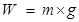and given in the question that value of g is greater at the poles than at the equator, hence weight of same amount of gold will be lesser at equator than it was on the poles. Therefore, the friend will not agree with the weight of gold bought.

###### 11. Why will a sheet of paper fall slower than one that is crumpled into a ball?

Ans. A greater surface area offers greater resistance and buoyancy same is true in the case of a sheet of paper that has larger surface area as compared to paper crumpled into a ball. So sheet of paper falls slower.

NCERT Solutions for Class 9 Science Gravitation part 2

###### 12. Gravitational force on the surface of the moon is only 1/6 as strong as gravitational force on the earth. What is the weight in newtons of a 10 kg object on the moon and on the earth?

Ans. value of gravity on earth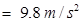value of gravity on moon = 1/6th of earth = 9.8/6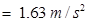weight of object on moon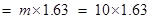= 16.3 N

weight of object on earth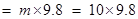= 98 N

###### 13. A ball is thrown vertically upwards with a velocity of 49 m/s. Calculate

(i) the maximum height to which it rises,

(ii) the total time it takes to return to the surface of the earth.

Ans. (i) v = u + gt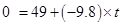9.8t = 49

t = 49/9.8 = 5 s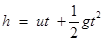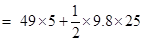= 245 – 122.5 = 122.5

(ii) total time taken to return = 5 + 5 = 10 s

###### 14. A stone is released from the top of a tower of height 19.6 m. Calculate its final velocity.

Ans.19.6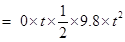4.9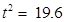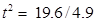= 4

t = 2

since v= u + at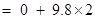= 19.6 m/s

###### 15. A stone is thrown vertically upward with an initial velocity of 40 m/s. Taking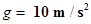, find the maximum height reached by the stone. What is the net displacement and the total distance covered by the stone?

Ans. Initial velocity of stone (u) = 40 m/s

at maximum height stone will be at rest so v= 0

v = u + gt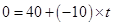10t = 40

t = 40/10 = 4 s

distance covered /maximum height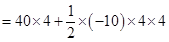= 160 – 80 = 80 m

net displacement of stone = 0(thrown upwards then falls back to same place)

total distance covered by the stone = 80 + 80 = 160 m

NCERT Solutions for Class 9 Science Gravitation part 2

###### 16. Calculate the force of gravitation between the earth and the Sun, given that the mass of the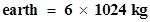and of the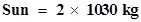. The average distance between the two is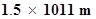.

Ans. F = G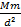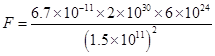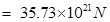###### 17. A stone is allowed to fall from the top of a tower 100 m high and at the same time another stone is projected vertically upwards from the ground with a velocity of 25 m/s. Calculate when and where the two stones will meet.

Ans. Suppose both the stones will meet after t seconds.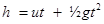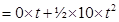= 5t2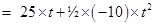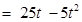h + h’ = 100 m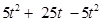= 100

25t = 100

t = 4 s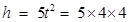= 80 m

Therefore, the two stones will meet after 4 seconds when the falling stone would have covered a height of 80 m.

NCERT Solutions for Class 9 Science Gravitation part 2

###### 18. A ball thrown up vertically returns to the thrower after 6 s. Find

(a) the velocity with which it was thrown up,

(b) the maximum height it reaches, and

(c) its position after 4 s.

Ans. (a)time taken by ball to reach maximum height(t) = 6/2 = 3 s

v = u + gt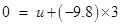u = 29.4 m/s (the velocity with which it was thrown up)

(b) the maximum height it reaches: therefore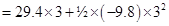= 88.2 – 44.1

= 44.1 m

(c) its position after 4 s will be:

Since in first 3 s it will reach the maximum height and in next 1 s it will start a free fall so, u = 0, t =1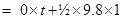= 4.9 m

therefore, after 4s the position of ball = 44.1 – 4.9 = 39.2 m

###### 19. In what direction does the buoyant force on an object immersed in a liquid act?

Ans. In the upward direction only.

###### 20. Why does a block of plastic released under water come up to the surface of water?

Ans. Since of plastic has density very less as compared to water i.e. weight of plastic is less than the buoyant force experienced by it therefore a block of plastic released under water come up to the surface of water/floats.

NCERT Solutions for Class 9 Science Gravitation part 2

###### 21. The volume of 50 g of a substance is 20 cm3. If the density of water is 1 g cm–3, will the substance float or sink?

Ans. Density of that substance (d) = mass/volume = 50/20 =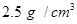since the density of substance (2.5) is greater than the density of water (1) therefore it will sink.

###### 22. The volume of a 500 g sealed packet is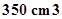. Will the packet float or sink in water if the density of water is 1 g cm–3? What will be the mass of the water displaced by this packet?

Ans. Density of the packet = mass/volume = 500/350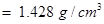Since the density of packet is more than density of water so it will sink. And packet will displace water equal to its volume :

volume of water displaced by packet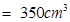(volume of packet)

mass of water displaced = volume of water displaced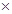density of water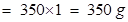## NCERT Solutions for Class 9 Science

NCERT Solutions Class 9 Science PDF (Download) Free from myCBSEguide app and myCBSEguide website. Ncert solution class 9 Science includes text book solutions .NCERT Solutions for CBSE Class 9 Science have total 15 chapters. 9 Science NCERT Solutions in PDF for free Download on our website. Ncert Science class 9 solutions PDF and Science ncert class 9 PDF solutions with latest modifications and as per the latest CBSE syllabus are only available in myCBSEguide.

## CBSE app for Class 9

To download NCERT Solutions for class 9 Science, Computer Science, Home Science,Hindi ,English, Maths Social Science do check myCBSEguide app or website. myCBSEguide provides sample papers with solution, test papers for chapter-wise practice, NCERT solutions, NCERT Exemplar solutions, quick revision notes for ready reference, CBSE guess papers and CBSE important question papers. Sample Paper all are made available through the best app for CBSE students and myCBSEguide website.